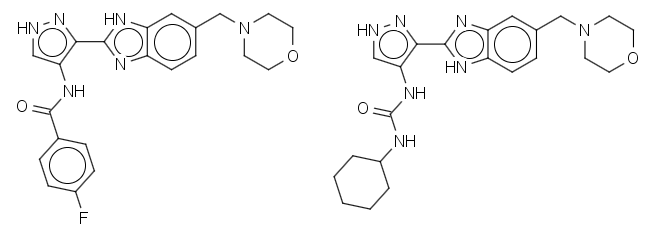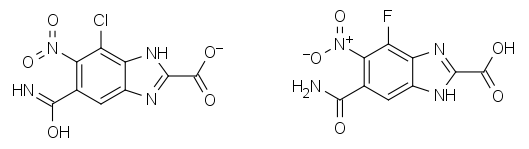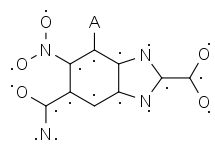# Fishing for matched series in a sea of structure representations

When searching for matched pairs/series, the typical approach is to use a fragmentation scheme and then collate the results for the same scaffold. Leaving aside other issues, we come to the question of how to ensure that all matched pairs for the same scaffold are actually found given the following representation issues: tautomeric forms (e.g. keto-enol), charge states (e.g. COO- versus COOH) and charge-separated/hypervalent forms (e.g. nitro as N(=O)=O or [N+]([O-])O).

Let’s take assay data in ChEMBL as an example. While the other issues are fairly well nailed down, the tautomer stored in ChEMBL is the first one encountered in the literature. This can lead to situations where the molecules from the same assay may have the same tautomer in the paper but not in ChEMBL (e.g. CHEMBL496754 and CHEMBL522563 from CHEMBL1009882):There are two approaches to sorting out these sorts of problems. The first is to try to generate a canonical representation of the molecule up-front. Note that this need not be the most preferred representation, just one that is canonical. An alternative approach is to create a hash for the structure that is invariant to representation issues and to use this hash to collate the scaffolds. This is actually quite a bit easier than the former approach. In an earlier blogpost, we described this method in the context of finding redox pairs, but it’s one of those ideas that bears repeating as it can be applied to several different problems.

I’ll call this method Sayle Hashing (after all, this fits with the nautical theme of the title). In this particular case, the Sayle Hash consists of two parts, a SMILES string and an integer. The integer is the total of the formal charges on the scaffold minus the number of hydrogens on each non-carbon atom, while the SMILES string is the canonical SMILES for the scaffold after setting all bond orders to 1 and hydrogen counts to 0. An example may be useful at this point. Here is a matched pair we would like to identify:Once fragmented at the halogen bond, we get the following non-identical scaffold SMILES:

```*c1c(c(C(=N)O)cc2nc([nH]c12)C(=O)[O-])N(=O)=O
*c1c(c(C(=O)N)cc2[nH]c(nc12)C(=O)O)[N+](=O)[O-]```

However, the corresponding Sayle Hashes are identical:

```*[C]1[C]([C]([CH][C]2[C]1[N][C]([N]2)[C]([O])[O])[C]([O])[N])N([O])[O]_4
*[C]1[C]([C]([CH][C]2[C]1[N][C]([N]2)[C]([O])[O])[C]([O])[N])N([O])[O]_4```Neat, huh? By the way, the values of 3 are from a hydrogen count of 3 and charge of -1, and a hydrogen count of 4 and charge of 0, respectively. This allows us to match these two scaffolds, arbitrarily picking one of the original representations to serve as the common scaffold.

## 3 thoughts on “Fishing for matched series in a sea of structure representations”

1.chemystry says:

Nice idea! But, does this deal with tautomers where one of the tautomeric forms has an H shifted from a heteroatom to a carbon atom, e.g. keto-enol tautomerism, enamine-imino tautomerism, or 1H- vs 3H-pyrrole (e.g. [nH]1cccc1 vs N1=CC([H])([H])C=C1)?

2.noel says:

Hi Steve. That’s right – (by definition) it only handles H shifts between hetereoatoms. But that’s already a big win for not a lot of work, given that it’s not constrained to 1,3- or 1,5- shifts. The same approach doesn’t easily extend to Hs on carbon; if done naively, Cl-Ph-cyclohexyl and F-cyclohexyl-Ph would be found as matched pairs!

1.chemystry says:

I thought so, but as you say, it is still a big win for a small cost

This site uses Akismet to reduce spam. Learn how your comment data is processed.